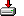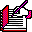#getzips.sas```%macro getzips (data=tables.zipcode, findzip=?, xmiles=, out=work.gotzips);

%local data findzip xmiles out;

%* Richard A. DeVenezia, Copyright 2000
%* Remsen, NY 13438
%* contact: http://www.devenezia.com/contact.php
%*
%* Coded:  9/25/00
%* Update: 4/ 2/04 fixed problem (reported by Zhonghe Li) with %if after first proc sql
%*
%* Purpose:
%*   Determine zipcodes within (<=) xmiles of zipcode findzip
%*   Distance is computed as 'spherical distance' using the Haversine Formula
%*
%* Related Topic:
%*   Calculate distance between two (2) points given latitude and longitude
%*
%* Arguments:
%*   data    - table of zip codes and 'centroid' location in latitude and longitude
%*   findzip - zipcode to which distances are computed
%*   xmiles  - zipcodes within this radius (miles) of zipcode are to be returned
%*   out     - output table that will contain only zipcodes
%*
%* Requirements:
%* zipdata
%*   zipcode column (char) must be named ZIPCODE
%*   latitude column (number) must be named LATITUDE and units degrees
%*   longitude column (number) must be named LONGTUDE and units degrees
%*
%* Caveats:
%*   No assumptions about centroid locations being projected or unprojected.
%*   Distance between points is 'surface distance', see
%*   http://earth.uni-muenster.de/~eicksch/GMT-Help/msg00147.html
%*   or search the web for "surface distance between points on map"
%*   or consult the GIS FAQ (http://www.census.gov/geo/www/faq-index.html)
%*   If a validation error occurs, the output data set is not touched
%*
%* Formula:
%*   Haversine Formula (from R.W. Sinnott, "Virtues of the Haversine",
%*   Sky and Telescope, vol. 68, no. 2, 1984, p. 159):
%*   dlon = lon2 - lon1
%*   dlat = lat2 - lat1
%*   a = sin^2(dlat/2) + cos(lat1) * cos(lat2) * sin^2(dlon/2)
%*   c = 2 * arcsin(min(1,sqrt(a)))
%*   d = R * c
%*   where
%*   lat and lon are latitude and longitude in radian respectively
%*   radians = degrees * pi / 180, (pi/180) = 0.017453293
%*   R = 3956, radius of Earth in miles
%*;

%let d2r = 0.017453293; %* radians / degree ;

%* validate zipcode data set;

%local zipCol latCol longCol;

%let zipCol = ZIPCODE;
%let latCol = LATITUDE;
%let longCol= LONGTUDE;

%local error dsid vn1 vn2 vn3 vt1 vt2 vt3;

%let error = 0;

%let dsid = %sysfunc (open (&data));
%if &dsid > 0 %then %do;
%let vn1 = %sysfunc (varnum (&dsid, &zipCol));
%let vn2 = %sysfunc (varnum (&dsid, &latCol));
%let vn3 = %sysfunc (varnum (&dsid, &longCol));

%if &vn1 = 0 %then %do;
%put ERROR: &data does not contain column &zipCol..;
%let error = 1;
%end;
%if &vn2 = 0 %then %do;
%put ERROR: &data does not contain column &latCol..;
%let error = 1;
%end;
%if &vn3 = 0 %then %do;
%put ERROR: &data does not contain column &longCol..;
%let error = 1;
%end;
%if &error %then %goto ByeBye;

%let vt1 = %sysfunc (vartype (&dsid, &vn1));
%let vt2 = %sysfunc (vartype (&dsid, &vn2));
%let vt3 = %sysfunc (vartype (&dsid, &vn3));

%if &vt1 ne C %then %do;
%put ERROR: column &zipCol is of type &vt1 and should be character.;
%let error = 1;
%end;
%if &vt2 ne N %then %do;
%put ERROR: column &latCol is of type &vt2 and should be numeric.;
%let error = 1;
%end;
%if &vt3 ne N %then %do;
%put ERROR: column &longCol is of type &vt3 and should be numeric.;
%let error = 1;
%end;
%if &error %then %goto ByeBye;

%let dsid = %sysfunc (close (&dsid));
%end;
%else %do;
%put ERROR: &data could not be opened.;
%let error = 1;
%end;
%if &error %then %goto ByeBye;

%* validate findzip;

%local zip1;

%let zip1 = %sysfunc (dequote (&findzip));

%if %length (&zip1) = %length (&findzip) %then %do;
%* findzip was not dequoted, assume only a number was passed;
%if &zip1 ne ? %then %do;
%let zip1 = %sysfunc (putn (&zip1, z5.));
%let zip1 = "&zip1";
%put WARNING: Argument FINDZIP=&findzip was converted to zipcode &zip1..;
%end;
%else %do;
%put ERROR: Argument FINDZIP= was not specified.;
%goto ByeBye;
%end;
%end;
%else %do;
%* findzip was dequoted, use original argument;
%let zip1 = &findzip;
%end;

%* obtain the latitude and longitude of findzip (and convert to radians);

%local lat1r long1r;

%let lat1r = -1;
%let long1r = -1;

Proc SQL noprint;
select min (&latCol) * &d2r, min (&longCol) * &d2r
into :lat1r, :long1r
from &data
where &zipCol = &findzip
having count(*) > 0;
quit;

%if %sysevalf(&lat1r = -1) or %sysevalf(&long1r = -1) %then %do;
%goto ByeBye;
%end;

%* select the zipcodes whose sphere surface distance is <= from zip1;

Proc SQL;
create table &out (keep=&zipCol) as
select
&zipCol
, &latCol  * &d2r as lat2r
, &longCol * &d2r - &long1r as dLon
, calculated lat2r - &lat1r  as dLat
, sin(calculated dLat/2)**2 as a1
, cos(&lat1r) as a2
, cos(calculated lat2r) as a3
, sin(calculated dLon/2)**2 as a4
,   calculated a1
+ calculated a2 * calculated a3 * calculated a4 as a
, 2 * arsin (min (1, sqrt(calculated a))) as c
, &radius * calculated c as distance
from &data
where
calculated distance <= &xmiles
;
;
quit;

%ByeBye:
%* make sure zipcode data set is closed when error exiting;
%if &dsid %then
%let dsid = %sysfunc (close (&dsid));

%mend getzips;

/*
%getzips (data=ctzip, findzip = "06013", xmiles = 25 );
*/```